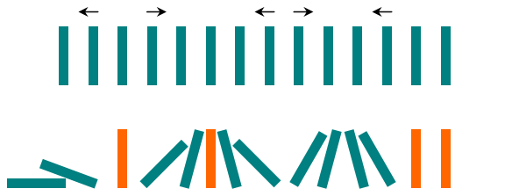## Algorithm

Problem Name: 838. Push Dominoes

There are `n` dominoes in a line, and we place each domino vertically upright. In the beginning, we simultaneously push some of the dominoes either to the left or to the right.

After each second, each domino that is falling to the left pushes the adjacent domino on the left. Similarly, the dominoes falling to the right push their adjacent dominoes standing on the right.

When a vertical domino has dominoes falling on it from both sides, it stays still due to the balance of the forces.

For the purposes of this question, we will consider that a falling domino expends no additional force to a falling or already fallen domino.

You are given a string `dominoes` representing the initial state where:

• `dominoes[i] = 'L'`, if the `ith` domino has been pushed to the left,
• `dominoes[i] = 'R'`, if the `ith` domino has been pushed to the right, and
• `dominoes[i] = '.'`, if the `ith` domino has not been pushed.

Return a string representing the final state.

Example 1:

```Input: dominoes = "RR.L"
Output: "RR.L"
Explanation: The first domino expends no additional force on the second domino.
```

Example 2:```Input: dominoes = ".L.R...LR..L.."
Output: "LL.RR.LLRRLL.."
```

Constraints:

• `n == dominoes.length`
• `1 <= n <= 105`
• `dominoes[i]` is either `'L'`, `'R'`, or `'.'`.

## Code Examples

### #1 Code Example with Java Programming

```Code - Java Programming```

``````
class Solution {
public String pushDominoes(String dominoes) {
int[] forces = new int[dominoes.length()];
int force = 0;
for (int i = 0; i < dominoes.length(); i++) {
if (dominoes.charAt(i) == 'R') {
force = dominoes.length();
} else if (dominoes.charAt(i) == 'L') {
force = 0;
} else {
force = Math.max(force - 1, 0);
}
forces[i] += force;
}
force = 0;
for (int i = dominoes.length() - 1; i >= 0; i--) {
if (dominoes.charAt(i) == 'L') {
force = dominoes.length();
} else if (dominoes.charAt(i) == 'R') {
force = 0;
} else {
force = Math.max(force - 1, 0);
}
forces[i] -= force;
}
StringBuilder result = new StringBuilder();
for (int i = 0; i < forces.length; i++) {
result.append(forces[i] > 0 ? 'R' : (forces[i] < 0 ? 'L' : '.'));
}
return result.toString();
}
}
``````
Copy The Code &

Input

cmd
dominoes = "RR.L"

Output

cmd
"RR.L"

### #2 Code Example with Python Programming

```Code - Python Programming```

``````start coding
class Solution:
def pushDominoes(self, dominoes):
res, l, r , pre_l, pre_r = "", {}, {}, None, None,
for i, s in enumerate(dominoes):
if s == "." and pre_r != None: r[i] = i - pre_r
elif s == "R": pre_r = i
elif s == "L": pre_r = None
for i in range(len(dominoes) - 1, -1, -1):
if dominoes[i] == "." and pre_l != None: l[i] = pre_l - i
elif dominoes[i] == "L": pre_l = i
elif dominoes[i] == "R": pre_l = None
for i, s in enumerate(dominoes):
if s == "L" or s == "R": res += s
elif i in l and i in r:
if l[i] < r[i]: res += "L"
elif r[i] < l[i]: res += "R"
else: res += s
elif i in l: res += "L"
elif i in r: res += "R"
else: res += s
return res
``````
Copy The Code &

Input

cmd
dominoes = "RR.L"

Output

cmd
"RR.L"